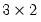Command Reference : Matrix Language Reference

 @mrnd
Matrix of uniform random numbers.
Syntax: @mrnd(n1, n2)
n1: integer
n2: integer
Return: matrix
Creates a matrix filled with uniform (0, 1) random numbers. The size of the matrix is given by the integers n1 (number of rows) and n2 (number of columns).
Examples
matrix m1 = @mrnd(3,2)
creates amatrix filled with uniform random numbers.
If the number of columns is 1, the function returns a vector, as in
vector v1 = @mrnd(18)
You may obtain a random sym by creating a non-square random normal and assigning the result to a sym matrix,
sym s1 = @mrnd(5, 5)
which assigns the main diagonal and below portion of the matrix to the sym S1.
Cross-references